Question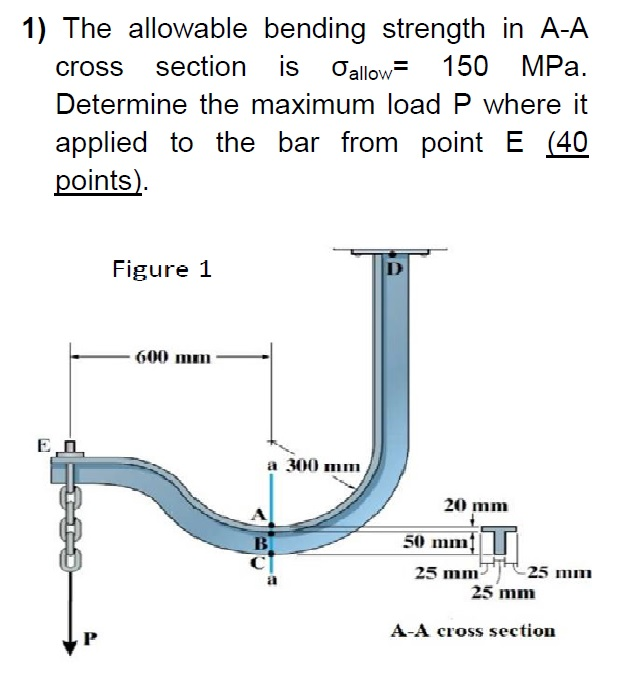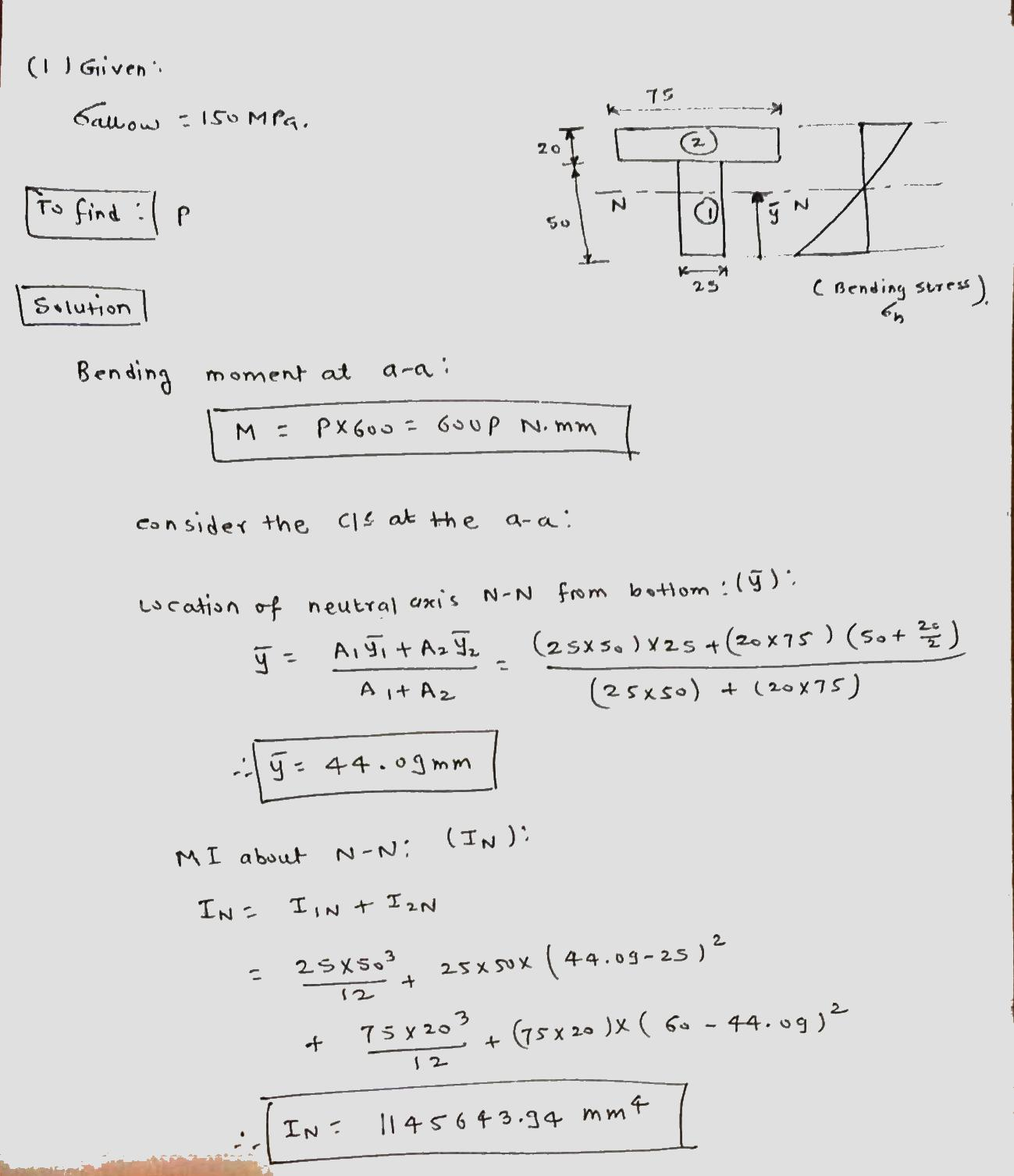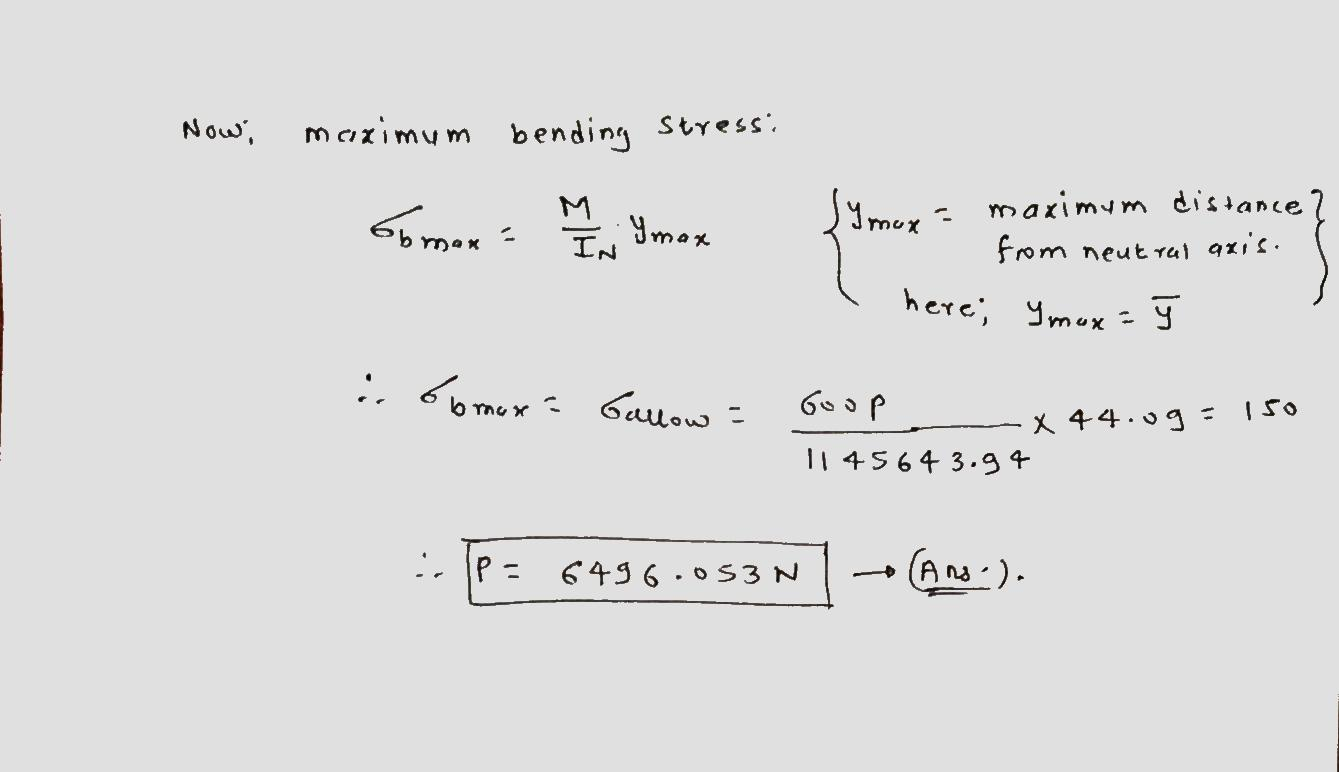Thank You!!!!

#### Earn Coins

Coins can be redeemed for fabulous gifts.

Similar Homework Help Questions
• ### The beam has the triangular cross section shown. The allowable stress in bending is 150 MPa....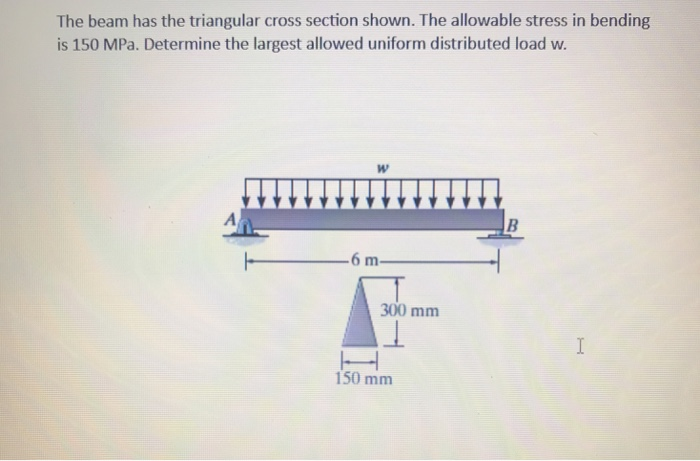The beam has the triangular cross section shown. The allowable stress in bending is 150 MPa. Determine the largest allowed uniform distributed load w. 6 m 300 mm 150 mm

• ### 1. Consider a simply-supported beam with a triangular cross section (Fig. 1). If the allowable bending...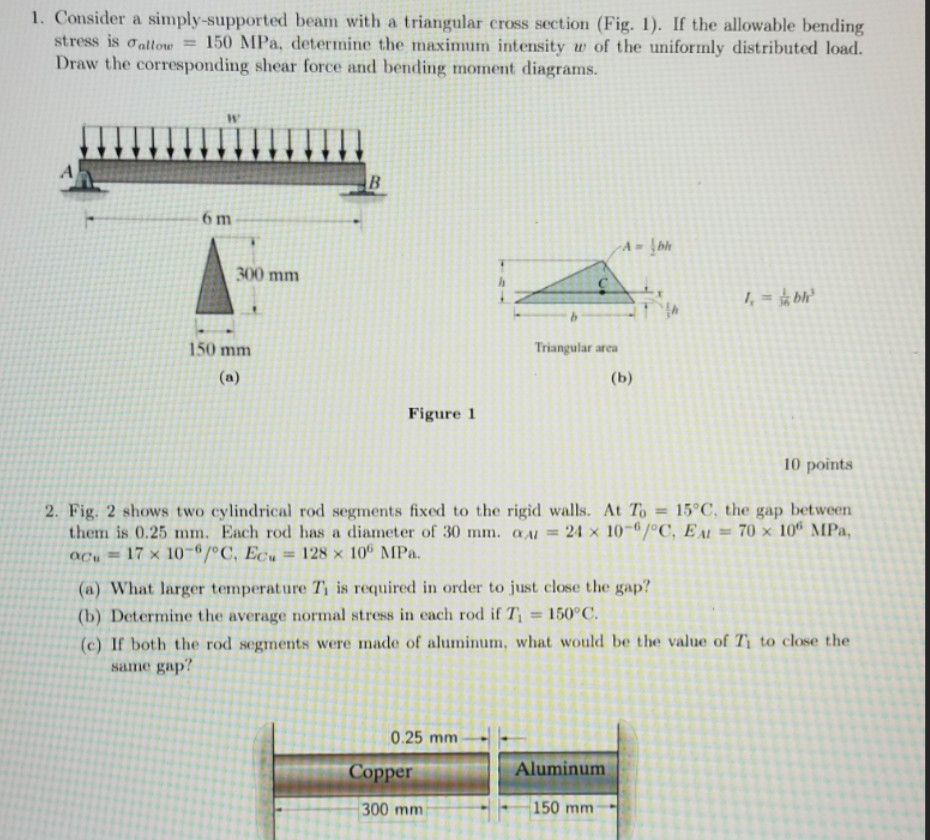1. Consider a simply-supported beam with a triangular cross section (Fig. 1). If the allowable bending stress is oallow = 150 MPa, determine the maximum intensity w of the uniformly distributed load. Draw the corresponding shear force and bending moment diagrams В 6m 300 mm 1bh 150 mm Triangular area (a) (b) Figure 1 10 points =15°C, the gap between 2. Fig. 2 shows two cylindrical rod segments fixed to the rigid walls. At To them is 0.25 mm. Each...

• ### Q4) If the allowable stress is 120 MPa in tension and 150 MPa in compression, determine...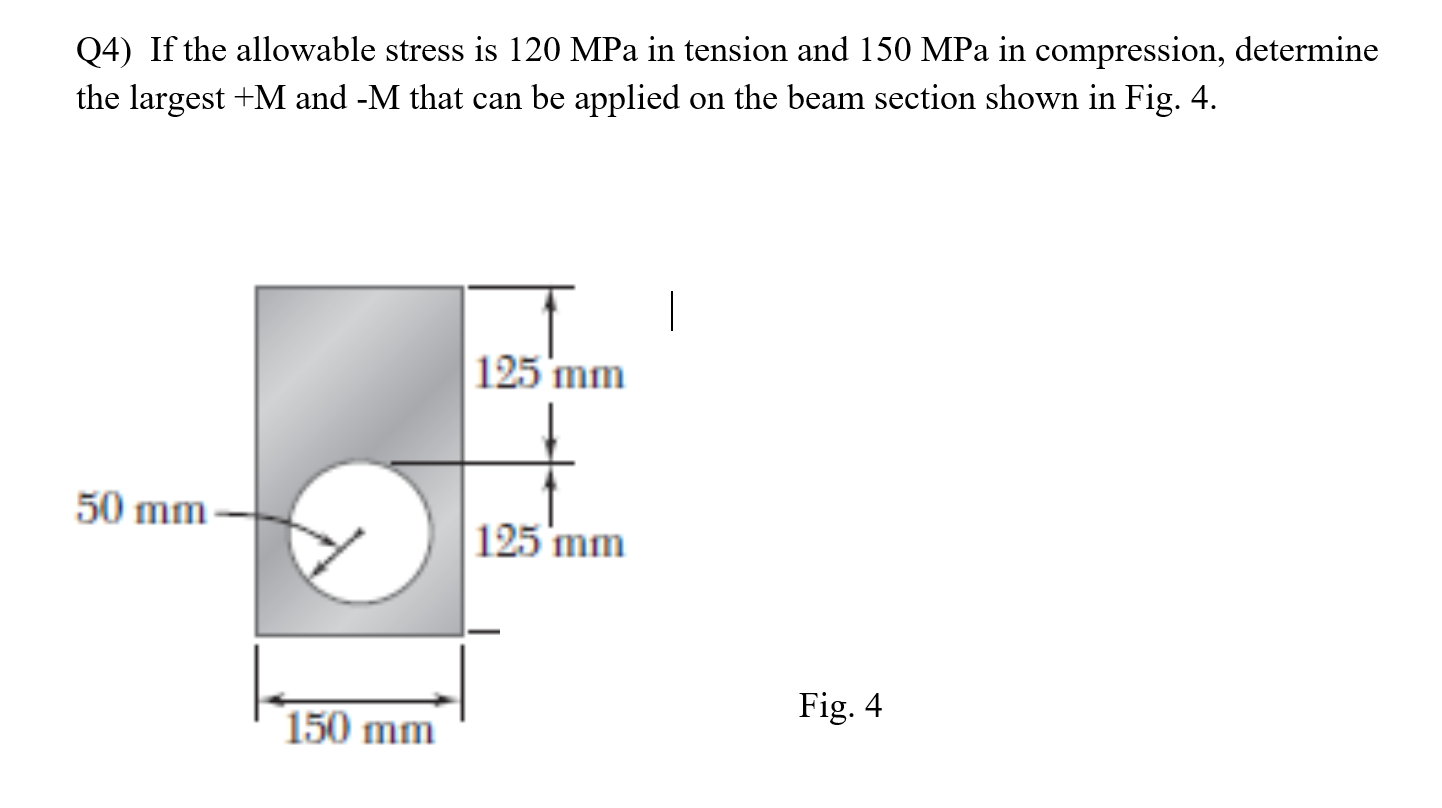Q4) If the allowable stress is 120 MPa in tension and 150 MPa in compression, determine the largest +M and -M that can be applied on the beam section shown in Fig. 4. 25 mm 50 mm 125 mm Fig. 4 150 mm

• ### Question #2 [12 marks] 15 mm The Figure shows a steel bar of rectangular cross section...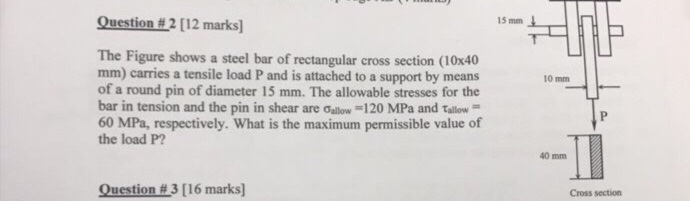Question #2 [12 marks] 15 mm The Figure shows a steel bar of rectangular cross section (10x40 mm) carries a tensile load P and is attached to a support by means of a round pin of diameter 15 mm. The allowable stresses for the bar in tension and the pin in shear are Gallow -120 MPa and Tallow 60 MPa, respectively. What is the maximum permissible value of 10 mm the load P? 40 mm Question #3 [16 marks] Cross...

• ### The bar has a 40 mm by 20 mm rectangular cross section. The allowable normal and...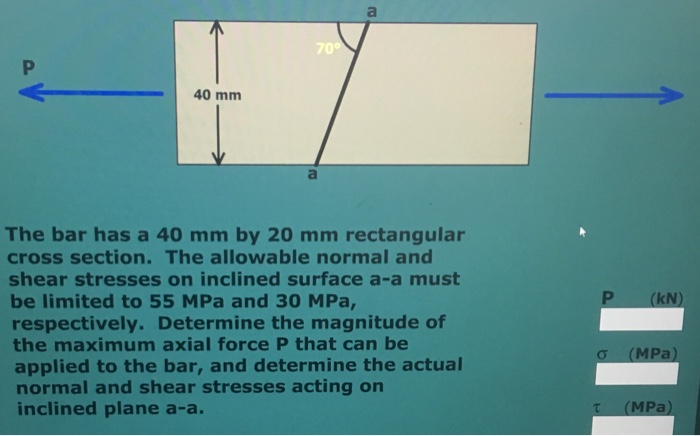The bar has a 40 mm by 20 mm rectangular cross section. The allowable normal and shear stresses on inclined surface a-a must be limited to 55 MPa and 30 MPa respectively. Determine the magnitude of the maximum axial force P that can be applied to the bar, and determine the actual normal and shear stresses acting on inclined plane a-a (MPa)

• ### Q5 Consider an extruded aluminium machine part that has the cross-section shown in the accompanying figure....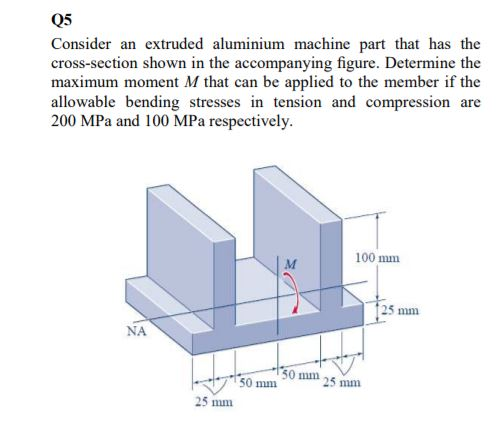Q5 Consider an extruded aluminium machine part that has the cross-section shown in the accompanying figure. Determine the maximum moment M that can be applied to the member if the allowable bending stresses in tension and compression are 200 MPa and 100 MPa respectively 100 mm 25 mm NA 50 mm 25 mm 50 mm 25 mm

• ### Strength of Material 3.7 A cantilever with a length of 1,5 m has a cross-section as...Strength of Material 3.7 A cantilever with a length of 1,5 m has a cross-section as shown in figure 3.16. Calculate: a) the magnitude of the allowable vertical load at the free end if the maximum allowable stress is 150 MPa, b) the deflection at the free end. E = 200 GPa. [2,97 kN; 13,57 mm) .8 12 112 80 ALERY Figure 3.16 A2 = 30 y los 69

• ### Problem 5 The cross-section shown below is subject to a positive internal bending moment M =...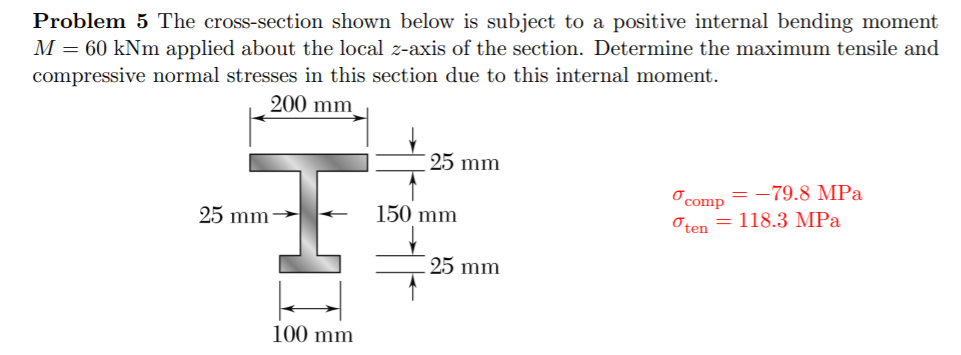Problem 5 The cross-section shown below is subject to a positive internal bending moment M = 60 kNm applied about the local z-axis of the section. Determine the maximum tensile and compressive normal stresses in this section due to this internal moment. 200 mm - 25 mm 25 mm 150 mm comp = -79.8 MPa O ten = 118.3 MPa 25 mm 100 mm

• ### QUESTION 1 Calculate the actual endurance strength in MPa, of cast steel bar having an endurance...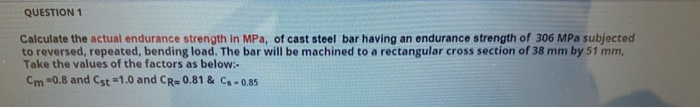QUESTION 1 Calculate the actual endurance strength in MPa, of cast steel bar having an endurance strength of 306 MPa subjected to reversed, repeated, bending load. The bar will be machined to a rectangular cross section of 38 mm by 51 mm, Take the values of the factors as below: Cm -0.8 and Cst =1.0 and CR=0.81 & Cs - 0.85

• ### Part B - Minimum Allowable Cross-Section The rod shown in the figure below is supported by...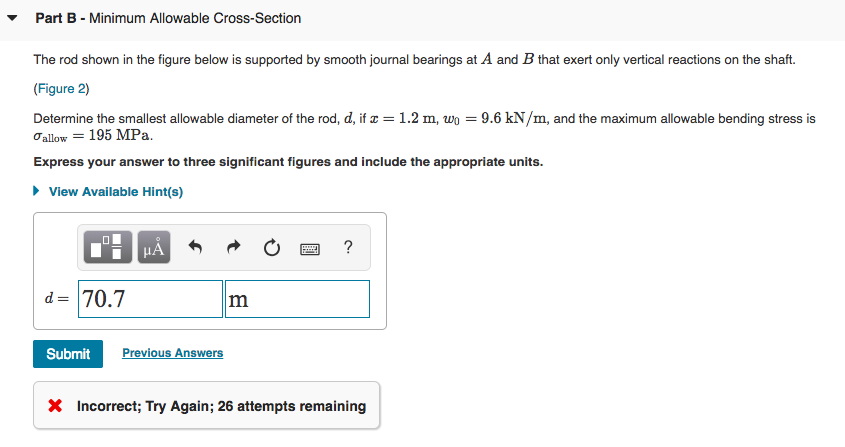Part B - Minimum Allowable Cross-Section The rod shown in the figure below is supported by smooth journal bearings at A and B that exert only vertical reactions on the shaft. (Figure 2) = 1.2 m, wo = 9.6 kN/m, and the maximum allowable bending stress is Determine the smallest allowable diameter of the rod, d, if Oallow = 195 MPa. Express your answer to three significant figures and include the appropriate units. ► View Available Hint(s) O 2 ?...